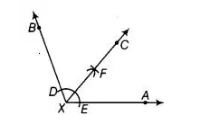# Draw an angle of 110° with the help`
Question:

Draw an angle of 110° with the help of a protractor and bisect it. Measure , each angle.

Solution:

Draw ∠BXA = 110° with the help of a protractor. Now, we use the following steps for required construction1. Taking X as centre and any radius daw an arc to Intersect the rays XA and XB, say at E and D, respectively.
2. Taking D and E as centres and with the radius more than ½ DE, draw arcs to intersect
each other, say at F.
3. Draw the ray XF.
Thus, ray XF is the required bisector of the angle B X A. On measuring each angle, we get
∠BXC = ∠AXC = 55°.
[∴ ∠BXC = ∠AXC = ½ ∠BXA = ½ x 110° = 55° ]